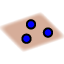# Planes viewed through a cube

Topic:
Cube, Planes
To create a plane through three points, click on the following icon:Examine the following questions.
1. How many points are in the image?  Explain.
2. How many segments are there between the 8 visible points?  Explain.
3. Determine whether or not there is a plane between the following points.
1. A, B, C, D
2. D, C, F, E
3. G, H, C, A
4. E, H, D
5. D, C, F
4. After completing this activity, what "shape" would you describe a plane to look like?  Why?